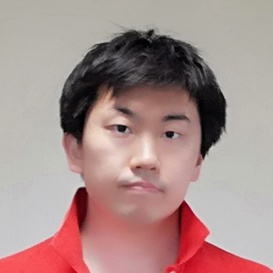atcoder

# # Problem

## # Input

$D G$
$p_1 c_1$
...
$p_D c_D$

• D - The number of ranks
• G - total score
• p_1 - The number of problems of rank i
• c_1 - The bonus score of rank i

The score of problem of rank i is $100 \times i$.

## # Output

The minimum number of problems to solve to arhieve a total score G or more.

# # Explanation

This problem can be solved by DFS about ranks in $O(2^D)$ as D is enough small.

Possible choice for each rank is either

1. Solve all the problems of the rank (with bonus)
1. Don't solve any problem of the rank
1. Solve problems of the rank partially (without bonus)

# # Time complexity

$O(2^D)$

# # Solution

#define MAX_D 11

struct Rank {
Int numProblems, score, bonus;

Rank(): numProblems(0), score(0), bonus(0) {}

Rank(Int n, Int s, Int b): numProblems(n), score(s), bonus(b) {
}
};

Int D, G, c, p;
Rank ranks[MAX_D];

struct State {
Int index = 0, numTaken = 0, score = 0, partialIndex = 0;

State() {
}

bool success() {
return score >= G;
}

bool end() {
return index >= D;
}

State takePart() {
State copy = *this;
Int need = (G - score) / ranks[index].score;
if (need > ranks[index].numProblems) return copy;
copy.score += ranks[index].score * need;
copy.numTaken += need;
return copy;
}

State take() {
State copy = *this;
copy.score += ranks[index].score * ranks[index].numProblems + ranks[index].bonus;
copy.numTaken += ranks[index].numProblems;
return copy;
}

State inc() {
State copy = *this;
copy.index++;
return copy;
}
};

void input() {
cin >> D >> G;
loop(d,1,D+1) {
cin >> p >> c;
ranks[d-1] = Rank(p, 100*d, c);
}
reverse(ranks, ranks+D);
}

State dfs(State state) {
if (state.success() || state.end()) return state;
if (state.takePart().success()) return state.takePart();

State not_take = dfs(state.inc());
State take = dfs(state.take().inc());
if (not_take.success() && take.success())
return (not_take.numTaken < take.numTaken) ? not_take : take;
if (take.success()) return take;
else return not_take;
}

void solve() {
State init;
State result = dfs(init);
cout << result.numTaken << endl;
}

int main(void) {
input();
solve();
return 0;
}Remote freelancer. A web and mobile application enginner.
Traveling around the world based on East Asia.
I'm looking forward to your job offers from all over the world!

Offer jobs or contact me!